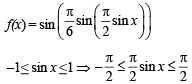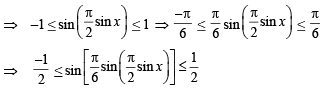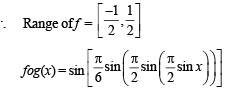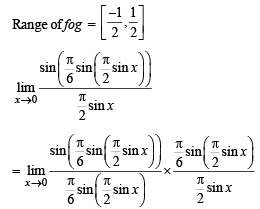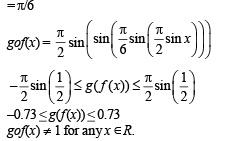Courses

# Test: MCQs (One or More Correct Option): Functions | JEE Advanced

## 11 Questions MCQ Test Maths 35 Years JEE Mains & Advance Past year Papers Class 12 | Test: MCQs (One or More Correct Option): Functions | JEE Advanced

Description
This mock test of Test: MCQs (One or More Correct Option): Functions | JEE Advanced for JEE helps you for every JEE entrance exam. This contains 11 Multiple Choice Questions for JEE Test: MCQs (One or More Correct Option): Functions | JEE Advanced (mcq) to study with solutions a complete question bank. The solved questions answers in this Test: MCQs (One or More Correct Option): Functions | JEE Advanced quiz give you a good mix of easy questions and tough questions. JEE students definitely take this Test: MCQs (One or More Correct Option): Functions | JEE Advanced exercise for a better result in the exam. You can find other Test: MCQs (One or More Correct Option): Functions | JEE Advanced extra questions, long questions & short questions for JEE on EduRev as well by searching above.
*Multiple options can be correct
QUESTION: 1

###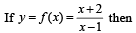Solution: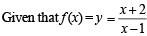Let us check each option one by one.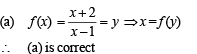(b) f (1) ≠ 3 as function is not defined for x = 1
∴ (b) is not correct.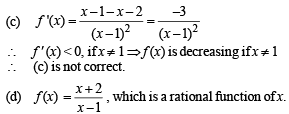*Multiple options can be correct
QUESTION: 2

### Let g (x) be a function defined on [– 1, 1]. If the area of the equilateral triangle with two of its vertices at (0,0) and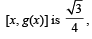then the function g(x) is

Solution:

As (0, 0) and (x, g (x)) are two vertices of an equilateral triangle; therefore, length of the side of D is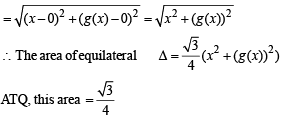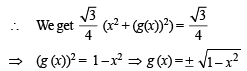∴ (b), (c) are the correct answers as (a) is not a function
(∴ image of x is not unique)

*Multiple options can be correct
QUESTION: 3

###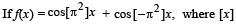stands for the greatest integer function, then

Solution:

f(x) = cos [π2] x + cos [– π2] x
We know 9 < π2 < 10 and – 10 < – π2 < – 9
⇒ [π2] = 9 and [–π2] = – 10
⇒ ∴ f (x) = cos 9x + cos (–10x)
f (x) = cos 9x  + cos 10 x
Let us check each option one by one.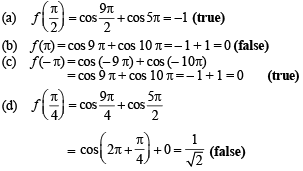Thus (a) and (c) are the correct options.

*Multiple options can be correct
QUESTION: 4

If f(x) = 3x – 5, then f–1(x)

Solution:

f (x) = 3x – 5 (given), which is strictly increasing on R,
so f –1 (x) exists.
Let y = f (x) = 3x – 5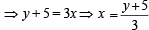...(1)
and y = f (x)  ⇒ x = f –1(y)   ...(2)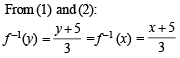*Multiple options can be correct
QUESTION: 5

If g (f(x)) = | sin x | and f (g(x)) = (sin √x)2, then

Solution:

Let us check each option one by one.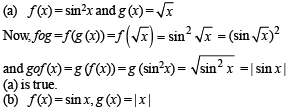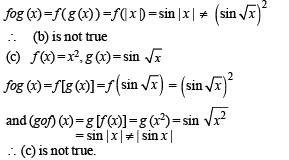*Multiple options can be correct
QUESTION: 6

Let f : (0, 1) → R be defined by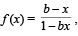where b is a constant such that 0 < b < 1. Then

Solution: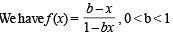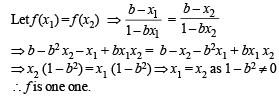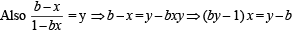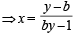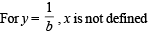∴ f is neither onto nor invertible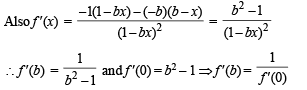Hence a and b are the correct options.

*Multiple options can be correct
QUESTION: 7

Let f : (–1, 1) ⇒ IR be such that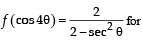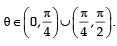Then the value (s) of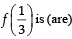Solution: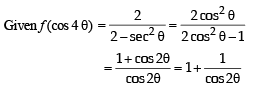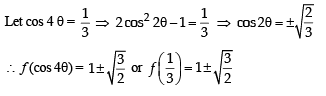*Multiple options can be correct
QUESTION: 8

The function f(x) = 2|x| + |x + 2| – | |x + 2| – 2 |x| has a local minimum or a local maximum at x =

Solution: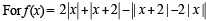the critical points can be obtained by solving |x| = 0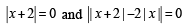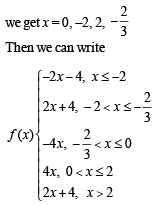The graph of y = f(x) is as follows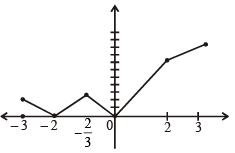From graph f(x) has local minimum at –2 and 0 and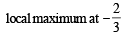*Multiple options can be correct
QUESTION: 9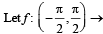R be given by f (x) = (log(sec x + tan x))3.

Then

Solution: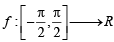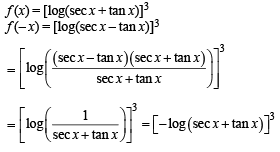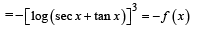∴ f  is an odd function.
(a) is correct and (d) is not correct.
Also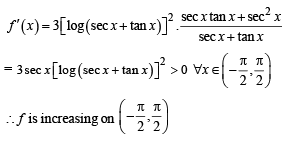We know that strictly increasing function is one one.
∴ f  is one one
∴ (b) is correct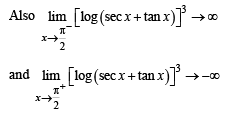∴ Range of f = (–∞, ∞) = R
∴ f  is an onto function.
∴ (c) is correct.

*Multiple options can be correct
QUESTION: 10

Let a ∈ R and let f : R → R be given by f (x) = x5 – 5x + a. Then

Solution:

f (x) = x5 - 5 x+a
f (x) = 0  ⇒ x5 - 5 x +a = 0
⇒ a = 5x – x5 = g(x)
⇒ g(x) = 0 when x = 0, 51/4 - 51/4 and g' (x ) = 0 ⇒ x = 1, – 1
Also g (– 1) = – 4 and g(1) = 4
∴ graph of g(x) will be as shown below.
From graph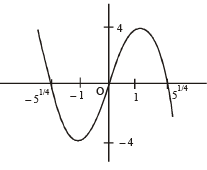if a ∈ ( -4,-4)
then g(x) = a or f (x) = 0 has 3 real roots If a > 4 or a < – 4
then f(x) = 0 has only one real root.
∴ (b) and (d) are the correct options.

*Multiple options can be correct
QUESTION: 11

Let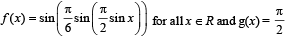sin x for all x ∈ R. Let (fog)(x) denote f(g(x)) and (gof)(x) denote g(f(x)). Then which of the following is (are) true?

Solution: